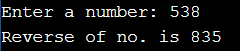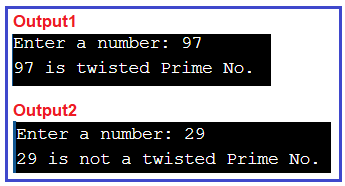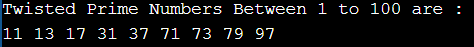# Twisted Prime Number in C#

## Twisted Prime Number in C# with Examples

In this article, I am going to discuss the Twisted Prime Number in C# with Examples. Please read our previous article where we discussed How to Implement Disarium Number Program in C#. Here, first, we will discuss what is a prime number, then we will discuss how to reverse a number, and finally, we will see how to Implement the Twisted Prime Number Program in C#.

##### Twisted Prime Number in C#

If the number and its reverse is a prime number then it is a twisted prime number. For example, 97 is a twisted prime number. Because 97 and if we reverse 97, i.e. 79 both are prime numbers.

Input: 97
Output: Twisted Prime Number
Explanation: 97 is a prime number and it’s reverse 79 is also a prime number.

Input: 43
Output: Not a Twisted Prime Number
Explanation: 43 is a prime number but it’s reverse 34 is not a prime number.

First of all, we have to know what is a prime number. How it works. What is the basic method to solve this problem?

##### What is a Prime Number?

It is a number that is greater than 1 and divided by 1 or itself only. For example, 2, 3, 5, 7, 11, 13, 17….
Here, we are going to use two operators: –

1) Modulus %: Modulus Operator will calculate the remainder after an integer division.
Example: 97 % 10 = 7
43 % 10 = 3
2) Divides /: Divides numerator by de-numerator
Example: 97 / 10 = 9
43 / 10 = 4

##### Prime Number Check

For this, you can visit our previous article where we have discussed prime number in great details with various examples

https://dotnettutorials.net/lesson/prime-numbers-in-csharp/

##### Twisted Prime Number Explanation

The idea is first to check if n is a prime number or not. If it is a prime number then reverse the number n and check reversed n is a prime number or not. We use basically three steps to solve this problem.

1. Check if a number is prime.
2. Then we will reverse the digits of that number.
3. Then after check, the reverse number is also a prime number or not. If the reverse number is also a prime number then it is a twisted prime number otherwise it is not a twisted prime number.

So, it is clear from our explanation above that for testing a number is a twisted prime number or not before that we need to learn that how to calculate the reverse of a number.

##### Reverse digit of a number in C#

The following program shows how to reverse a number in C#.

```using System;
class ReverseDigit
{
public static void Main ()
{
int revNo = 0;
Console.Write ("Enter a number: ");

//Calcuating reverse of a number.
while (num > 0)
{
revNo = revNo * 10 + num % 10;
num = num / 10;
}
Console.Write ("Reverse of no. is " + revNo);
}
}
```
###### Program Explanation:

This program takes an integer input from the user. Then the while loop is used until n != 0 is false (0). In each iteration of the loop, the remainder when n is divided by 10 is calculated and the value of n is reduced by 10 times. Inside the loop, the reversed number is computed using:

revNo = revNo * 10 + num % 10;

Output: When you execute the above program, you will get the following outputAfter understanding how to reverse our number let’s go to our real program which twisted prime number.

##### Twisted Prime Number in C#
```using System;
{
public static void Main ()
{
Console.Write ("Enter a number: ");
int num = Convert.ToInt32 (Console.ReadLine ());

//Calclating Reverse of Number
int revNo = ReverseNo(num);

//Condition check for both the number (Entered number and Reversed No)
//if both are prime then it is twisted prime number.
if (IsPrime (num) && IsPrime (revNo))
Console.WriteLine (num + " is twisted Prime No.");
else
Console.WriteLine (num + " is not a twisted Prime No.");
}

//Method to calculate reverse of number
public static int ReverseNo(int num)
{
int revNo = 0;
while (num > 0)
{
revNo = revNo * 10 + num % 10;
num = num / 10;
}
return revNo;
}

//Method to check for prime number
public static bool IsPrime(int num)
{
bool isPrime = true;
for (int i = 2; i < num / 2; i++)
{
if (num % i == 0)
{
isPrime = false;
break;
}
}
return isPrime;
}
}
```
###### Output:##### Twisted Prime Numbers between 10 to 100 in C#:

Now lets us see how to print all the twisted prime numbers between 10 to 100.

```using System;
{
public static void Main ()
{
Console.Write ("Twisted Prime Numbers Between 1 to 100 are : \n");

for(int i = 10; i <= 100; i++)
{
//Calclating Reverse of Number
int revNo = ReverseNo(i);

//Condition check for both the number (Entered number and Reversed No)
//if both are prime then it is twisted prime number.
if (IsPrime (i) && IsPrime (revNo))
Console.Write(i + " ");
}

}

//Method to calculate reverse of number
public static int ReverseNo(int num)
{
int revNo = 0;
while (num > 0)
{
revNo = revNo * 10 + num % 10;
num = num / 10;
}
return revNo;
}

//Method to check for prime number
public static bool IsPrime(int num)
{
bool isPrime = true;
for (int i = 2; i < num / 2; i++)
{
if (num % i == 0)
{
isPrime = false;
break;
}
}
return isPrime;
}
}
```
###### Output:In the next article, I am going to discuss How to Implement Buzz Number Program in C# with Examples. Here, in this article, I try to explain How to Implement the Twisted Prime Number Program in C# and I hope you enjoy this Twisted Prime Number Program in the C# article.